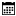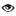#### AutoMapper

Rakesh Kumar Sutar |12 January 2021 |1375

## Introduction

C# Supports conditional statement. There are 4 types of conditional statement.
- IF statement
- ELSE statement
- ELSE...IF statement
- SWITCH Statement

IF statement is used when some code need to be execute for a specific condition is true.

ELSE statement is used when some code need to be execute for a specific condition is false.

ELSE... IF statement is used when first condition is false and some other condition need to be tested.

SWITCH statement is used when to specify many alternative blocks of code to be executed.

## Syntax

IF statement

if (condition)
{
// block of code to be executed if the condition is True
}

ELSE statement

if (condition)
{
// block of code to be executed if the condition is True
}
else
{
// block of code to be executed if the condition is False
}

ELSE... IF statement

if (condition1)
{
// block of code to be executed if condition1 is True
}
else if (condition2)
{
// block of code to be executed if the condition1 is false and condition2 is True
}
else
{
// block of code to be executed if the condition1 is false and condition2 is False
}

## Example - IF statement

```using System;

namespace Hello_world
{
class Program
{
static void Main(string[] args)
{
int a = 10;
int b = 20;
if(a>b)
{
Console.WriteLine(a   " is Greater");
}

if(b>a)
{
Console.WriteLine(b   " is Greater");
}
}
}
}
```## Example - ELSE statement

```using System;

namespace Hello_world
{
class Program
{
static void Main(string[] args)
{
int a = 10;
int b = 20;
if(a>b)
{
Console.WriteLine(a   " is Greater");
}

else
{
Console.WriteLine(b   " is Greater");
}
}
}
}

```

## Output## Example - ELSE... IF statement

```using System;

namespace Hello_world
{
class Program
{
static void Main(string[] args)
{
int a = 10;
int b = 20;
int c = 30;
if(a>b)
{
Console.WriteLine(a   " is Greater");
}

else if(c>a)
{
Console.WriteLine(c   " is Greater");
}
else
{
Console.WriteLine(b  " is Greater");
}
}
}
}

```

## Output